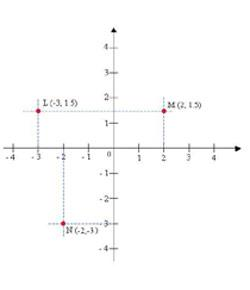# Coordinate Geometry Act Questions

15 Questions | Total Attempts: 427SettingsCoordinate Geometry ACT Questions

• 1.
In the standard (x,y) coordinate plane below, the points (0,2), (8,2), (3,6) and (11,6) are teh vertices of a parallelogram. What ist he area, in square units, of the parallelogram?
• A.

8.49

• B.

16

• C.

32

• D.

56

• E.

88

• 2.
What is the approximate distance between the points (4, -3) and (-6, 5) in the standard (x, y) coordinate plane?
• A.

8.92

• B.

12.81

• C.

16.97

• D.

17.95

• E.

19.22

• 3.
Given the vertices of parallelogram FGHJ in the standard (x, y) coordinate plane below, what is the area of triangle GHJ in square units?
• A.

11

• B.

15

• C.

22

• D.

44

• E.

88

• 4.
In the figure above, OS = ST and the coordinates of T are (k, 5). What is the value of k?
• A.

-5

• B.

-3

• C.

-2

• D.

0

• E.

5

• 5.
What is the slope of a line that is perpendicular to the line determined by the equation 5x + 8y = 17?
• A.

-3

• B.

-5/8

• C.

17/8

• D.

3/17

• E.

8/5

• 6.
In the standard (x, y) coordinate plane shown below, what is the distance on the y-axis, in units, from point A to point B?
• A.

-3

• B.

-5

• C.

3

• D.

5

• E.

11

• 7.
An overlay of an accessibility ramp of a building is placed on the standard (x,y) coordinate plane so that the x-axis aligns with the horizontal. The line segment representing the side view of the ramp goes through the point (-2, -1) and (16, 2). What is the slope of the accessibility ramp?
• A.

-3

• B.

-1/3

• C.

-1/6

• D.

1/6

• E.

1/14

• 8.
In the standard (x, y) coordinate plane, point B with coordinates (5, 6) is the midpoint of AC, and A has coordinates (6, 7). What are the coordinates of C?
• A.

(11, 13)

• B.

(7, 8)

• C.

(4, 5)

• D.

(5.5, 6.5)

• E.

(-4, -8)

• 9.
In the square graphed below, what is the slope of the line segment AC?
• A.

4

• B.

2

• C.

1

• D.

-1

• E.

-4

• 10.
Which of the following is the slope of a line parallel to the line y = 2/5x + 7 in the standard (x, y) plane?
• A.

-7

• B.

-5/2

• C.

2/5

• D.

2

• E.

5/2

• 11.
Quadrilateral QRST is shown below in the standard (x, y) coordinate plane.What is the length of QS in coordinate units?
• A.

A

• B.

B

• C.

C

• D.

D

• E.

E

• 12.
Which of the following is closest to the perimeter of quadrilateral QRST, in coordinate units?
• A.

26.0

• B.

22.5

• C.

19.8

• D.

15.0

• E.

14.0

• 13.
What is the slope-intercept form of 10x - y - 8 = 0?
• A.

Y = -2x

• B.

Y = -10x - 8

• C.

Y = -10x + 8

• D.

Y = 10x - 8

• E.

Y = 10x + 8

• 14.
What is the distance in standard (x, y) coordinate plane between the points (5, 5) and (1, 0)?
• A.

A

• B.

B

• C.

C

• D.

D

• E.

E

• 15.
What is the slope of the line determined by the equation 2x - 3y = 6?
• A.

-6

• B.

-3

• C.

-3/2

• D.

2/3

• E.

2

Related TopicsBack to top